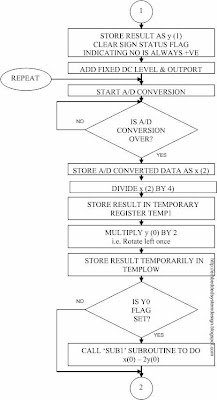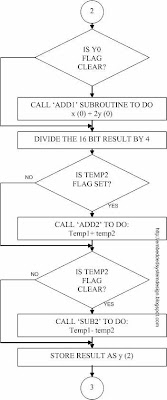## 31 May 2009

### IMPLEMENTATION OF CHEBYSHEV TYPE –1(ORDER-2) BANDPASS FILTER

Filter Parameters:

Order, 2n = 2

Passband = 450Hz - 550Hz.

Sampling Frequency Fs = 2000Hz

Using “fdatool” of MATLAB software the obtained filter coefficient (truncated to 4th decimal point) are

b = [0.2374, 0, 0.2374]

a = [1, 0, 0.5]

These coefficients are scaled by a factor 4 and again truncated to give filter coefficients as,

b = [1, 0, 1 ]

a = [4, 0, 2 ]

Linear Difference equation representation: - From the filter coefficients we can write the transfer function as

H (z)= [1+0.Z-1-1.Z-2] / [4+0.Z-1+2.Z-2 ]

Converting above equation to linear difference equation form we have,

4y(n)+2(n-2) = x (n)-x (n-2)

y (n) = 1/4[x (n)-x (n-2)-2y(n-2)]

For n = 0, y (0) = 1/4[x (0)]…………………………….(1)

For n = 1, y (1) = 1/4[x (1)]…………………………….(2)

For n = 2, y (2) = 1/4[x (2)]-1/4[x (0)+2y(0)]…………..(3)

Hardware Implementation And Analysis:

All the hardware setup exercised in previous study of filter implementation remains the same. To implement this filter only software has to be changed.

All the discussion carried over the sampling frequency effect, noise rejection ability of the filter etc hold for this filter also. Thus the discussion and analysis of this filter is going to be a repetition of what we have said in earlier section instead let us look into the peculiar problem that we have faced in implementing this filter and how work around is sorted out to solve the problem.

Keenly observe the equation (3), we can observe that at one instance the value of 1/4[x (2)] becomes less than 1/4[x (0)+2y(0)] when this happens, the total result y (2) comes out to be a –ve value. How PIC can understand that it is a –ve data? It treats each data as a +ve binary number and accordingly processes it. Then how to come out of this mischievous loop?

There is one more problem, which is peeping out! The problem is after some looping of the software and assignment of present value to previous value .We may get y(0) values as –ve. At this junction, ¼[x (0)+(-2y(0)] may result a –ve output. Now we have to handle one more –ve number, which the PIC unaware of!

To get through all these obstacles we have set a flag bit to indicate the sign status of the each resultant output y0, y1 and y2 (of course, then as you know, it loops). If the number is –ve, the flag bit is set else it is cleared.

Now let us imagine that y (0) is –ve. Then 2y(0) will be a –ve number. Then instead of adding x (0) with 2y(0) (refer equation (3)), we should subtract 2y(0) from x(0). This result also may be a –ve number. To indicate sign condition of this temporary result one more flag bit ‘ tempflag’ is used. According to the sign condition of this ‘tempflag’, 1/4[x (0)+2y(0)] is either added with or subtracted from 1/4[x (2)]. In the case of subtraction once again the result may come out to be a –ve value. To eliminate this –ve value a fixed DC level is added to each output before it finally out ported to PIC ports. The fixed DC value is selected such a way that it is greater than or equal to the resultant maximum –ve value.

Initially to check the numbers are –ve or +ve numbers are simply subtracted and checked for borrow. If carry is borrowed number from which another number is subtracted is treated as a –ve number (by setting corresponding flag bit), else it is treated as +ve number (by clearing corresponding flag bits). When present output value is assigned to previous output value, corresponding flag condition is also assigned accordingly.

FLOWCHARTS:

MAIN PROGRAM:SUBROUTINE ‘SUB1’:SUROUTINE ‘SUB2’:SUBROUTINE ‘ADD1’:SUROUTINE ‘ADD2’:SUBROUTINE ‘DELAY’:References

1. “Digital Signal Processing” By Sanjit K.Mitra

2. “Digital Signal Processing” By P.Ramesh Babu

3. “Digital Filters” By T.J.Terrel and E.T.Powner

4. “BASIC Digital Signal Processing” By Gordon B. Lockart and Barry M.G.Cheetham

5. “Digital Signal Processing” By Alan V.Oppenheim and Ronald W.Schafer

6. “DSP Microprocessors: Advances and Automotive Applications” By Subra Ganeshan and Dr.Gopal Arvamudhan

7. PIC 16F87XA Data Sheet

8. Embedded Control Handbook-1994/95/Microchip

9. Microchip Technical Library CD-ROM-First Edition 2002

10. National Semiconductor CD-ROM-1995/96

11. “Design with PIC Microcontrollers” By John B.Peatman

12. “Opamps and Linear Integrated Circuits” By Ramakant A.Gayakwad

13. “Electronic Principles” By A.P.Malvino

#### Post a Comment

Your Comments... (comments are moderated)